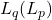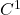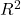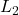# Publications

1. (with Kijung Lee and Jinsol Seo) A weighted Sobolev regularity theory on the parabolic equations with measurable coefficients on conic domains, Journal of  Differential Equations 291(2021), 154-194 (pdf)
2. (with Daehan Park and Junhee Ryu) An-theory for diffusion equations with space-time nonlocal operators.  Journal of Differential Equations 287 (2021), 376-427 (pdf)
3. (with Beom-seok Han and Daehan Park) A weighted Sobolev space theory for the diffusion-wave equations with time-fractional derivatives ondomains. Discrete and Continuous Dynamical System 41 (2021), no. 7, 3415-3445 (pdf)
4. (with Kijung Lee and Jinsol Seo) A refined Green’s function estimate of the time measurable parabolic operators with conic domains. (pdf) to appear in Potential Analysis
5. (with Beom-seok Han) Boundary behavior and interior Holder regularity of solution to nonlinear stochastic partial differential equations driven by space-time white noise.   Journal of Differential Equation 269 (2020), 9904-9935. (pdf)
6. (with Beom-seok Han and Daehan Park) Weighted-estimate with Muckenhoupt weights for the diffusion-wave equations with time-fractional derivative.  Journal of Differential Equations 269 (2020), 3515-3550. (pdf)
7. (with Ildoo Kim) Boundedness of stochastic singular integral operators and its application to stochastic partial differential equations.  Transactions of AMS 373 (2020),  5653-5684 (pdf)
8. (with P.A. Cioica-Licht and Kijung Lee) On the regularity of the stochastic heat equation on polygonal domains in.  Journal of Differential Equations 267 (2019), 6447-6479. (pdf)
9.  (with I.Kim and S.Lim) A Sobolev space theory for stochastic partial differential equations with time-fractional derivatives.  Annals of Probability 47 (2019), no 4, 2087-2139. (pdf)
10. (with I.Kim and P.Kim) An-theory for diffusion equations related to stochastic processes with non-stationary independent increment.  Transactions of AMS. 371 (5) (2019), 3417-3450.  (pdf)
11. (with I.Kim) On the second order derivative estimates for degenerate parabolic equations.  Journal of Differential Equations 265 (2018), 5959-5930. (pdf)
12. (with I.Kim) A regularity theory for quasi-linear stochastic partial differential equations in weighted Sobolev space. Stochastic processes and their applications 128(2018) 622-643.  (pdf)
13. (with P. Cioica, K.Lee and F. Lindner) An-estimate for the stochastic heat equation on an angular domain in.  Stochastics and Partial Differential Equations: analysis and computations.  6(1) (2018), 45-72. (pdf)
14. (with K. Lee).  On the heat diffusion starting with degeneracy. Journal of Differential Equations 262(2017), no.3, 2722-2744. (pdf)
15. (with I. Kim and S. Lim). An-theory for the time fractional evolution equations with variable coefficients.  Advances in  Math. 306 (2017), 123–176. (pdf
16. (with I. Kim and S. Lim). An-theory for parabolic pseudo-differential equations: Calderón-Zygmund approach. Potential Anal. 45 (2016), no. 3, 463–483. (pdf
17. (with I. Kim). An-theory for stochastic partial differential equations driven by Lévy processes with pseudo-differential operators of arbitrary order.  Stochastic Process.  Appl.126 (2016), no. 9, 2761–2786. (pdf)
18. (with S. Lim). Asymptotic behaviors of fundamental solution and its derivatives to fractional diffusion-wave equations.  J. Korean Math. Soc.53 (2016), no. 4, 929–967. (pdf
19. (with K. Lee). A weighted-theory for second-order parabolic and elliptic partial differential systems on a half space. Commun.  Pure Appl. Anal.15 (2016), no. 3, 761–794. (pdf
20. (with I. Kim and S. Lim). Parabolic Littlewood-Paley inequality for a class of time-dependent pseudo-differential operators of arbitrary order, and applications to high-order stochastic PDE.  J. Math. Anal. Appl.436 (2016), no. 2, 1023–1047. (pdf)
21. (with I. Kim). An-theory for a class of non-local elliptic equations related to nonsymmetric measurable kernels.  J. Math. Anal. Appl.434 (2016), no. 2, 1302-1335 (pdf)
22.  (with I. Kim). A Hölder regularity theory for a class of non-local elliptic equations related to subordinate Brownian motions.  Potential Anal.43 (2015), no. 4, 653–673. (pdf)
23. (with I. Kim and S. Lim). Parabolic BMO estimates for pseudo-differential operators of arbitrary order.  J. Math. Anal. Appl. 427 (2015), no. 2, 557–580. (pdf)
24. (with Z.Q.Chen and P. Kim). Fractional time stochastic partial differential equations.  Stochastic Process. Appl.125 (2015), no. 4, 1470–1499. (pdf)
25. (with Z.Q. Chen). An L_p-theory for non-divergence form SPDEs driven by Lévy processes.  Forum Math.26 (2014), no. 5, 1381–1411. (pdf)
26. (with I. Kim).  Someand Hölder estimates for divergence type nonlinear SPDEs on-domains.  Potential Anal. 41 (2014), no. 2, 583–612. (pdf)
27. A weighted Sobolev space theory of parabolic stochastic PDEs on non-smooth domains. Journal Theoret. Probab.27 (2014), no. 1, 107–136. (pdf)
28. (with I. Kim and K. Lee).  A weighted-theory for divergence type parabolic PDEs with BMO coefficients on-domains.  J. Math. Anal. Appl.412 (2014), no. 2, 589–612. (pdf)
29. A Sobolev space theory for parabolic stochastic PDEs driven by Lévy processes on-domains.  Stochastic Process. Appl.124 (2014), no. 1, 440–474. (pdf)
30. (with I.Kim and P. Kim). Parabolic Littlewood-Paley inequality for-type operators and applications to stochastic integro-differential equations.   Advances in Mathematics. 249 (2013), 161–203. (pdf)
31. (with P. Cioica, K. Lee and F. Lindner). On the-regularity and Besov smoothness of stochastic parabolic equations on bounded Lipschitz domains.  Electron. J. Probab.18 (2013), no. 82, 41 pp. (pdf)
32.  (with K. Lee). A-theory of stochastic parabolic partial differential systems on-domains.  Potential Anal. 38 (2013), no. 3, 951–984.
33. (with K. Lee). A weighted-theory for parabolic PDEs with BMO coefficients on-domains.  J. Differential Equations.  254(2013), no.2, 368-407. (pdf)
34. (with K. Lee)A note on-theory of linear stochastic parabolic partial differential systems. Stochastic Process. Appl. 123 (2013), no. 1, 76–90. (pdf)
35. (with Z.Q. Chen)An-theory for a class of SPDEs driven by Lévy processes. Sci. China Math. 55 (2012), no. 11, 2233–2246.   (pdf)
36. (with P. Kim)An-theory of a class of stochastic equations with the random fractional Laplacian driven by Lévy processes. Stochastic Process. Appl. 122 (2012), no. 12, 3921–3952.  (pdf)
37. (with K. Lee) A-theory of elliptic and parabolic partial differential systems in-domains.  J. Math. Anal. Appl. 391 (2012), no. 2, 397–414  (pdf)
38. (with I. Kim). A generalization of the Littlewood-Paley inequality for the fractional Laplacian.  J. Math. Anal. Appl. 388 (2012), no. 1, 175–190.  (pdf)
39. (with K. Lee). A-theory of stochastic partial differential systems of divergence type on-domains. Electron.  J. Probab. 16 (2011), no. 47, 1296–1317.
40. (with C.H.Lee and P. Kim). A Moment closure method for stochastic reaction networks.  J. Chemical Physics. 130, 134107(2009).  (pdf)
41. Sobolev space theory of SPDEs with continuous or measurable leading coefficients. Stochastic Process. Appl. 119 (2009), no. 1, 16–44. (pdf)
42. A-theory of parabolic equations with unbounded leading coefficients on non-smooth domains.  J. Math. Anal. Appl. 350 (2009), no. 1, 294–305.  (pdf)
43. An-theory of stochastic PDEs of divergence form on Lipschitz domains. J. Theoret. Probab. 22 (2009), no. 1, 220–238. (pdf)
44. An-theory of SPDEs on Lipschitz domains. Potential Anal. 29 (2008), no. 3, 303–326. (pdf), (erratum)
45.theory of parabolic PDEs with variable coefficients.  Bull. Korean Math. Soc. 45 (2008), no. 1, 169–190. (pdf)
46.-theory of parabolic SPDEs degenerating on the boundary of-domains.  J. Theoret. Probab. 21 (2008), no. 1, 169–192. (pdf)
47. Sobolev space theory of parabolic equations degenerating on the boundary of-domains.  Comm. Partial Differential Equations 32 (2007), no. 7-9, 1261–1280. (pdf)
48. Parabolic SPDEs degenerating on the boundary of non-smooth domain. Electron. J. Probab. 11 (2006), no. 23, 563–584.  (pdf)
49.estimates for SPDE with discontinuous coefficients in domains. Electron. J. Probab. 10 (2005), no. 1, 1–20. (pdf)
50. (with N.V.Krylov). On the Sobolev space theory of parabolic and elliptic equations in-domains.  SIAM J. Math. Anal. 36 (2004), no. 2, 618–642. (pdf)
51.  On-theory of stochastic partial differential equations of divergence form in-domains. Probab.  Theory Related Fields 130 (2004), no. 4, 473–492.  (pdf)
52.-theory and Hlder estimates for parabolic SPDEs. Stochastic Process. Appl. 114 (2004), no. 2, 313–330. (pdf)
53. (with  N. V. Krylov). On SPDEs with variable coefficients in one space dimension. Potential Anal. 21 (2004), no. 3, 209–239. (pdf)
54. On stochastic partial differential equations with variable coefficients in-domains. Stochastic Process. Appl. 112 (2004), no. 2, 261–283. (pdf)